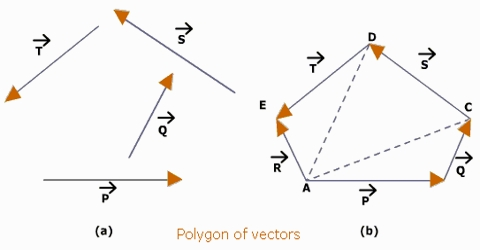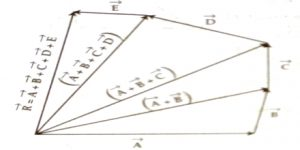# Law of Polygon in Geometrical Addition of Vector Quantities

Law of Polygon

This law states that if a vector polygon he drawn, placing the tail-end of each succeeding vector at the head or arrow-end of the preceding one, their resultant is drawn from the tail-end of the first to the head- or arrow-end of the last. If a number of vectors can be represented both in magnitude and direction by the sides of an open convex polygon taken in the same order, then the resultant is represented totally in magnitude and direction by the closing side of the polygon, taken in the opposite order.Explanation: Let us consider vectors; A, B, C, D, E [Fig] and their resultant vector is to be found out. Now, we place on the head or arrow-end of the first vector. A the tail-end of the vector B. Then on the head- or arrow-end of B the tail-end of C and following the same procedure other vectors are placed successively [Fig.]. Now connect the tail-end of vector A and the head- or arrow-end of the last vector i.e., E. Then, according to the law of polygon, this connecting vector R will be the resultant;

R = A + B + C + D + E